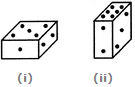# Non Verbal Reasoning - Cubes and Dice - Discussion

### Discussion :: Cubes and Dice - Section 2 (Q.No.9)

9.

Two positions of a parallelepiped are shown below. When the number 3 will be on the top side, then which number will be at the bottom?[A]. 1 [B]. 4 [C]. 5 [D]. 6

Explanation:

The number 2 is common to both the figures. We assume the parallelepiped in fig. (ii) to be rotated so that 2 appears at the same position as in fig. (i) i.e. on the RHS face and the numbers 6 and 3 move to the faces hidden behind the numbers 1 and 5 respectively [in fig. (i)]. Then, the combined figure will have 1 opposite 6 and 5 opposite 3. Thus, when 3 will be on the top, then 5 will appear at the bottom.

 Joiker34 said: (Feb 14, 2017) The explanation is kind of complicated. If you just look at where the three is, the bigger side in II and in I on the big side is 5. So 5 is opposite 3.